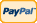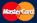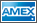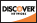Online Store Software Catalog and Price Product Registration Services News About Us Contact Us My Account Español
•Electronics, electrical engineering
•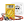Engineering physics
•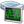Generators
•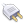Interfaces
•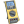Multimeters
•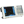Oscilloscopes
•Persona
•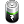Power supplies
•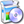Software
•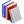Standards
•Thesaurus
•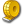Units of measurement
Filter by first letter
A
B
C
D
E
F
G
H
I
J
K
L
M
N
O
P
Q
R
S
T
U
V
W
X
Y
Z
+
-

# True-RMS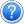Search:

As a rule in AC measuring process we are interested in the value equivalent to DC which action would cause the same thermal effect as the action of AC under measurement. This value is called current root-mean-square value (or RMS).

To get such value the following formula should be used: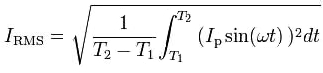The simplest way to measure such RMS with a measurement device is to rectify AC, to identify the rectified signal average value and to multiply the result by 1.1 ratio (average to ideal sine curve rms).

Although when the sine curve deviates from the ideal form, this ratio stops acting. Therefore measurement instruments with readings averaging often display wrong results in the process of current measurement in modern power mains.

Linear loads, which contain only resistors, coils and capacitors, can be characterized with the current sine curve that is why their parameters measurement never causes any problems. However in the case of nonlinear loads, such as adjustable frequency drive and power supplies for office equipment, there can appear distorted curves as a result of possible noises caused by powerful loads.

Current rms value measurement using such distorted curves with simple measurement instruments can show significant understating of true results depending on load factors:

 Device class Load type / curve forms Linear load (sine curve) PWM (meander) Single-phase diode rectifier Three-phase diode rectifier RMS correct overstating by 10% understating by 40% understating by 5%...30% True RMS correct correct correct correct

That is why users of simple measurement devices have a question why, for example, fuse of 14 A is regularly burnt out though according to the amperemeter indication the current is 10 A only.

To measure the current with distorted curves it’s necessary to check the sine curve form with a waveform analyzer, afterwards to use a measurement instrument with readings averaging but only in the case of the curve being ideal sine. But its much more convenient to use a measurement instrument (e.g. digital multimeter) with True RMS and be always sure that the measurement results are correct. Modern multimeters and clamp meters of such class use improved measurement technology which let them identify true effective AC value regardless of the curve being ideal sine or distorted. Therefore there are special converters used which set conditions for the main price difference between professional multimeters and budget multimeters. The only limitation is that the curve should be located within the accepted measurement range and the operating frequency range of the device used. Everything that concerns the characteristics of nonlinear load current measurement is correct for voltage measurement as well. Stress-strain curves are often not ideal sine curves, as a result of it measurement instruments (multimeters) with readings averaging display wrong results.

Back to the list

Units Converter
• How it Works
• Company News
• Industry News & Events
• Measurement Fun Facts